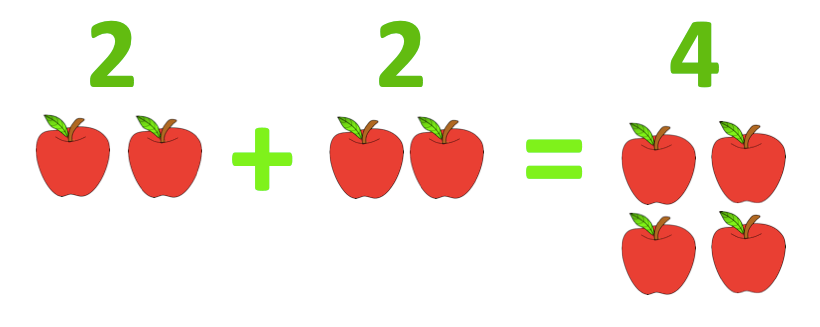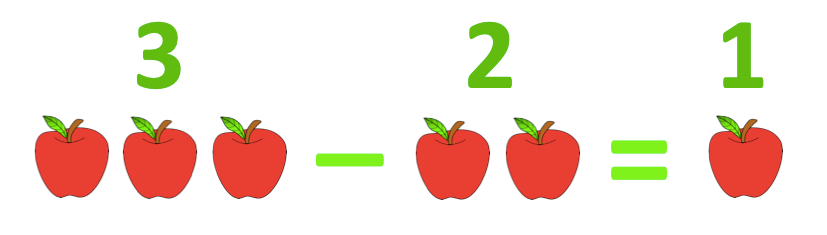Addition is a mathematical process in which you can find out the total or sum of a set of numbers. The symbol for addition is ‘+’.

What is two add two? Think of this as two apples and two apples, the total is four apples.For example:

2 + 2 = 4

4 + 3 = 7

6 + 4 = 10

8 + 1 = 9

5 + 7 = 12

8 + 10 = 18

14 + 5 = 19

# What is subtraction?

Subtraction is a mathematical process in which you can find the difference between two values, or take away. The symbol for subtraction is ‘-‘.

For basic subtraction, you can use your finger to count. For larger numbers, you can use beads or items to help you subtract.

What is three minus two? Think of this as three apples take away two apples, the total is one apple.For example:

4 – 2 = 2

6 – 3 = 3

7 – 1 = 6

9 – 5 = 4

10 – 2 = 8

14 – 7 = 7

16 – 5 = 11

Addition is a mathematical process in which you can find out the total or sum of a set of numbers. The symbol for addition is ‘+’.

What is two add two? Think of this as two apples  and two apples, the total is four apples.

🍎🍎 + 🍎🍎 = 🍎🍎🍎🍎

2      +    2     =          4

For example:

2 + 2 = 4

4 + 3 = 7

6 + 4 = 10

8 + 1 = 9

5 + 7 = 12

8 + 10 = 18

14 + 5 = 19

# What is subtraction?

Subtraction is a mathematical process in which you can find the difference between two values, or take away. The symbol for subtraction is ‘-‘.

For basic subtraction, you can use your finger to count. For larger numbers, you can use beads or items to help you subtract.

What is three minus two? Think of this as three apples take away two apples, the total is one apple.

🍎🍎🍎 – 🍎🍎 = 🍎

3        –    2     =   1

For example:

4 – 2 = 2

6 – 3 = 3

7 – 1 = 6

9 – 5 = 4

10 – 2 = 8

14 – 7 = 7

16 – 5 = 11